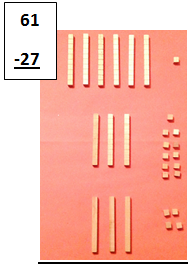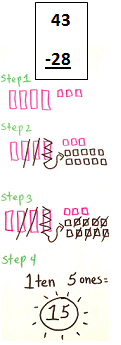# How to Borrow in Math

Instructor: Audrey Akins

Audrey has more than a decade of experience teaching elementary. She has a bachelor's in journalism and a master's in education.

After you learn to subtract, you will have experiences when you'll have to subtract two, or three-digit numbers using borrowing. In this lesson, we'll look at how to borrow using concrete objects, representational pictures, and abstract numbers.

## Borrowing Using Concrete Objects

Borrowing can be used for any place value. You will sometimes have to borrow from the tens place, the hundreds place, the thousands place, and even larger place values. For this lesson, we'll use the ones and tens places to subtract two-digit numbers. When we use concrete objects, this means we are using actual objects we can hold in our hands to compose and decompose the numbers we're working with. When we borrow, we are actually breaking the number in the place to the left.

For example, in the problem 61 - 27, since you can't subtract 7 from 1, you have to break a stick of ten or borrow a stick of ten from the tens place. Once you do that, you'll have 5 tens. Then, you'll move that stick of ten to the ones place so now you have 11 ones. Now you can subtract. 11 - 7 = 4. 5 - 2 = 3. So if you have 3 in the tens place and 4 in the ones place, the answer to 61 - 27 is 34. This is what it looks like to subtract with base ten blocks.## Using Pictures to Subtract

Once you are comfortable using base ten blocks to borrow when you are subtracting, you'll soon be able to do the same thing drawing pictures. If you have 43 rocks in your collection and sell 28 of them, you'll have to borrow to subtract. You can draw 4 tens and 3 ones with base ten blocks. Next, in order to subtract, borrow a stick of ten from the 4, which will leave you with 3 tens. Then add the stick of ten to the 3 ones, which gives you 13. You are now ready to subtract. 13 - 8 = 5. 3 - 2 =1. If you have 1 ten and 5 ones, your answer is 15.To unlock this lesson you must be a Study.com Member.

### Register to view this lesson

Are you a student or a teacher?